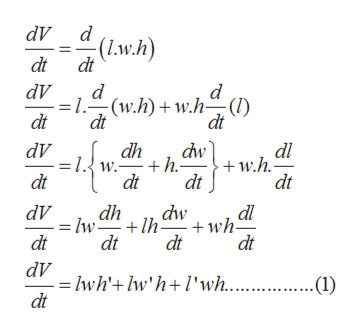# The length ℓ, width w, and height h of a box change with time. At a certain instant, the dimensions are ℓ = 6 m and w = h = 3 m, and ℓ and w are increasing at a rate of 1 m/s while h is decreasing at a rate of 9 m/s. At that instant find the rates at which the following quantities are changing.a) The volume.b) The surface area.c) The length of the diagonal. (Round answer to two decimal places)

Question
660 views

The length ℓ, width w, and height h of a box change with time. At a certain instant, the dimensions are ℓ = 6 m and w = h = 3 m, and ℓ and w are increasing at a rate of 1 m/s while h is decreasing at a rate of 9 m/s. At that instant find the rates at which the following quantities are changing.

a) The volume.

b) The surface area.

c) The length of the diagonal. (Round answer to two decimal places)

check_circle

Step 1

It is given that, the length(l), width(w) and height(h) of a box change with time(t).

And at a certain instant, length(l)=6m, width(w)= 3m and height(h)=3m.

Also,

Step 2

To find the rate at which the following quantities are changing at time(t).

• Volume
• Surface Area
• Length of the diagonal
Step 3
• The rate of change of the volum...help_outlineImage TranscriptionclosedV d (.w.h) dt dt dV d =1.-(w.h)w.h-(I) dt d dt dt dw h. dt dV dh W. dt dl w.h. dt dt dl dwwh- dV dh lw +lh dt dt dt dt = lwh'+lw'h+ l'wh... dt .(1) fullscreen

### Want to see the full answer?

See Solution

#### Want to see this answer and more?

Solutions are written by subject experts who are available 24/7. Questions are typically answered within 1 hour.*

See Solution
*Response times may vary by subject and question.
Tagged in

### Calculus